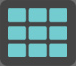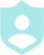# Fractions, Percents, and Decimals###### Example QuestionsWrite each as a decimal. Round to the thousandths place. 77.3%Write each as a decimal. Round to the thousandths place. 55.2%
##### Description :
In this game, you will answer questions on converting between fractions, decimals, and percents##### Difficulty :GRID 6 Categories 30 QuestionsPrintShareAdd
Questions
Number of categoriesQuestions per categoryCategory 1
Percent to DecimalCategory 2
Percent to FractionCategory 3
Decimal to PercentCategory 4
Decimal to FractionCategory 5
Fraction to PercentCategory 6
Fraction to DecimalLayout Preview
 Question Q. Write each as a decimal. Round to the thousandths place. 77.3% A. 0.773 Question Q. Write each as a fraction. 46.5% A. 93/100 Question Q. Write each as a percent. Round to the nearest tenth of a percent. 0.42 A. 42% Question Q. Write each as a fraction. 0.5 A. 1/2 Question Q. Write each as a percent. Round to the nearest tenth of a percent. 7/65 A. 10.8% Question Q. Write each as a decimal. Round to the thousandths place. 17/100 A. 0.17 Question Q. Write each as a decimal. Round to the thousandths place. 55.2% A. 0.552 Question Q. Write each as a fraction. 91.5% A. 183/200 Question Q. Write each as a percent. Round to the nearest tenth of a percent. 0.08 A. 8% Question Q. Write each as a fraction. 0.99 A. 99/100 Question Q. Write each as a percent. Round to the nearest tenth of a percent. 4/5 A. 80% Question Q. Write each as a decimal. Round to the thousandths place. 22/35 A. 0.629 Question Q. Write each as a decimal. Round to the thousandths place. 83.6% A. 0.836 Question Q. Write each as a fraction. 75% A. 3/4 Question Q. Write each as a percent. Round to the nearest tenth of a percent. 0.982 A. 98.2% Question Q. Write each as a fraction. 0.125 A. 1/8 Question Q. Write each as a percent. Round to the nearest tenth of a percent. 1/2 A. 50% Question Q. Write each as a decimal. Round to the thousandths place. 1/8 A. 0.125 Question Q. Write each as a decimal. Round to the thousandths place. 30.4% A. 0.304 Question Q. Write each as a fraction. 12.5% A. 1/8 Question Q. Write each as a percent. Round to the nearest tenth of a percent. 0.02 A. 2% Question Q. Write each as a fraction. 0.875 A. 7/8 Question Q. Write each as a percent. Round to the nearest tenth of a percent. 1/100 A. 1% Question Q. Write each as a decimal. Round to the thousandths place. 3/19 A. 0.158 Question Q. Write each as a decimal. Round to the thousandths place. 53% A. 0.53 Question Q. Write each as a fraction. 34.3% A. 343/1000 Question Q. Write each as a percent. Round to the nearest tenth of a percent. .7 A. 70% Question Q. Write each as a fraction. 0.332 A. 83/250 Question Q. Write each as a percent. Round to the nearest tenth of a percent. 22/25 A. 88% Question Q. Write each as a decimal. Round to the thousandths place. 7/10 A. 0.7
Colors :
Font color
Button color
Background color
Logo:Background Image:Theme Song: